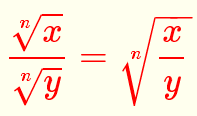Grade 10 questions on how to divide radical expressions with solutions are presented.

Quotient (Division) of Radicals With the Same Index

Division formula of radicals with equal indices is given byExamples

Simplify the given expressionsUse the above division formula to simplify the following expressionsSolutions to the Above Problems